# I) Project Analytics

Each project have an analytics section. Depending on how you are using Project Boards, you can see those reports:

## User Repartition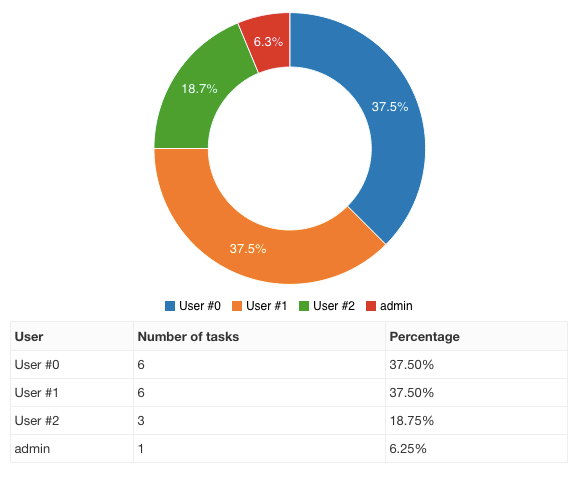This pie chart show the number of open tasks assigned per user.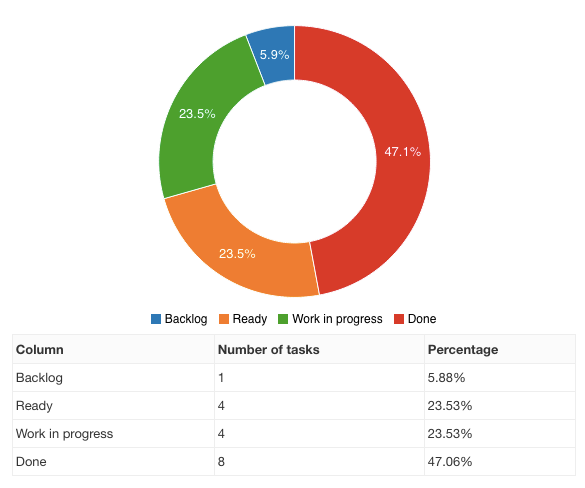This pie chart gives an overview of the number of open tasks per column.

## Cumulative Flow Diagram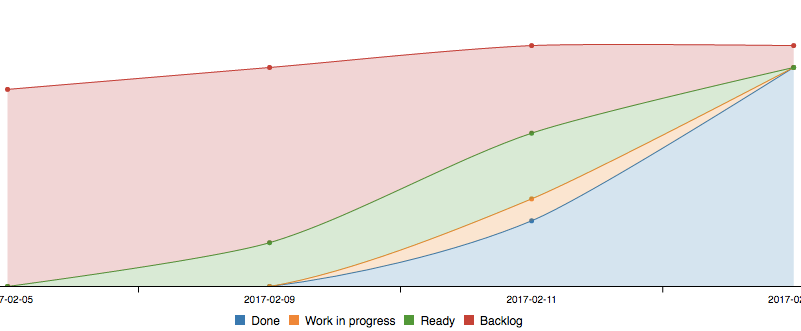• This chart shows the number of tasks cumulatively for each column over the time.
• The legend order is the same as the stack in the chart.
• The color of each column is determined automatically.
• Every day, the number of tasks is recorded for each column.
• If you would like to exclude closed tasks, change the global project settings.

Note: You need to have at least two days of data to see the graph.

## Burn Down Chart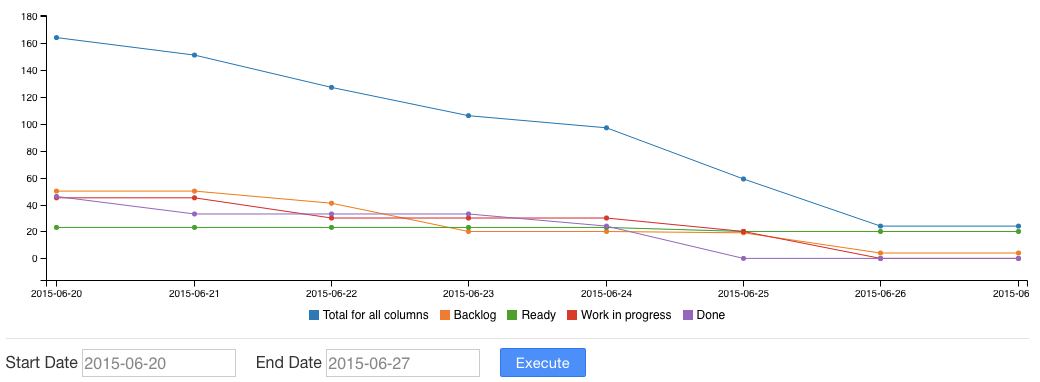The burn down chart is available for each project.

• This chart is a graphical representation of work left to do versus time.
• Project Boards use the complexity or story point to generate this diagram.
• Everyday, the sum of the story points for each column is calculated.

## Average Time Spent Into Each Column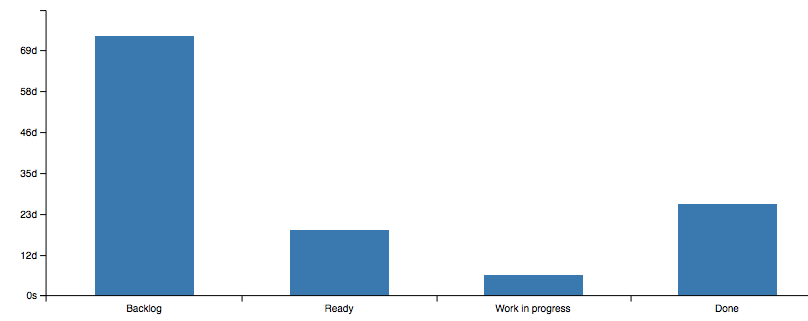This chart shows the average time spent into each column for the last 1000 tasks.

• Project Boards uses the task transitions to calculate the data.
• The time spent is calculated until the task is closed.

## Average Lead and Cycle time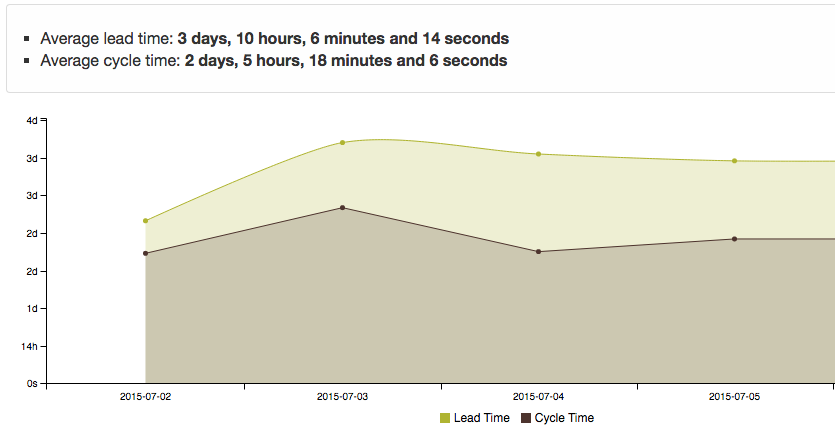This chart show the average lead and cycle time for the last 1000 tasks over time.

• The lead time is the time between the task creation and the date of completion.
• The cycle time is time between the specified start date of the task to the completion date.
• If the task is not closed, the current time is used instead of the date of completion.

Those metrics are calculated and recorded every day for the whole project.